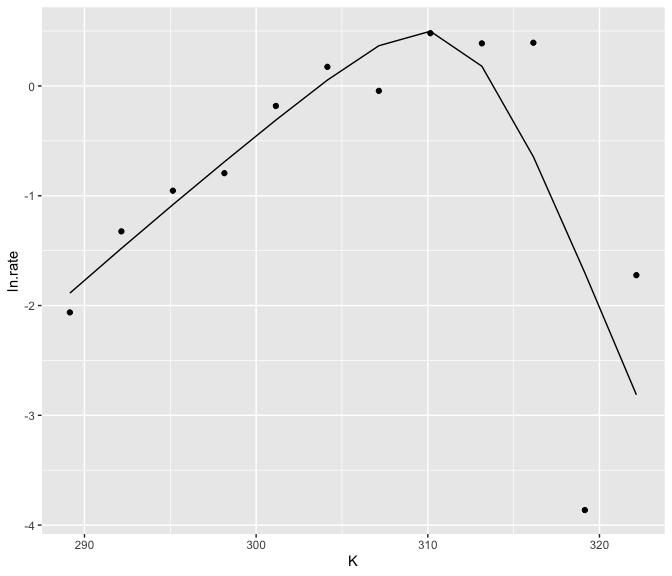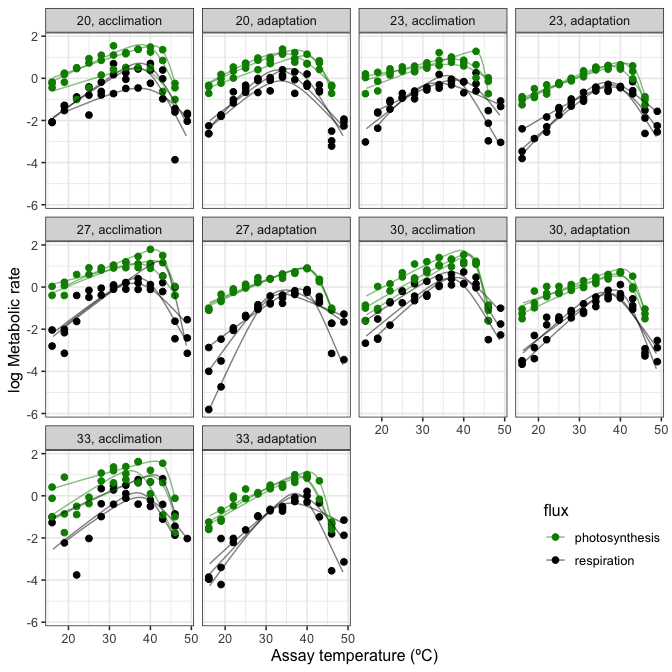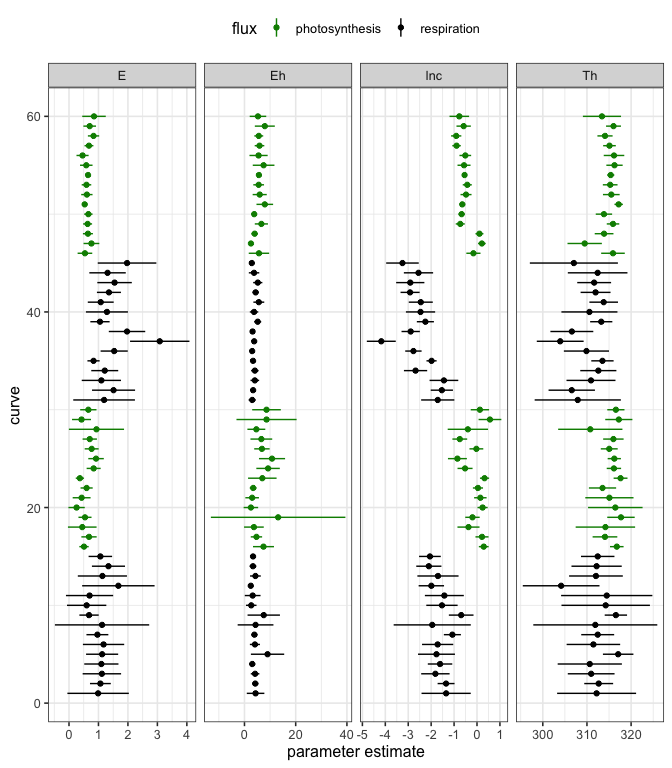Robust Non-Linear Regression using AIC Scores

Non-linear least squares regression with the Levenberg-Marquardt algorithm using multiple starting values for increasing the chance that the minimum found is the global minimum.

nls.multstart

Robust and reproducible non-linear regression in R

Granville Matheson: [email protected]

Issues and suggestions

Please report any issues/suggestions for improvement in the issues link for the repository. Or please email [email protected] or [email protected].Overview

nls.multstart is an R package that allows more robust and reproducible non-linear regression compared to nls() or nlsLM(). These functions allow only a single starting value, meaning that it can be hard to get the best estimated model. This is especially true if the same model is fitted over the levels of a factor, which may have the same shape of curve, but be much different in terms of parameter estimates.

nls_multstart() is the main (currently only) function of nls.multstart. Similar to the R package nls2, it allows multiple starting values for each parameter and then iterates through multiple starting values, attempting a fit with each set of start parameters. The best model is then picked on AIC score. This results in a more reproducible and reliable method of fitting non-linear least squares regression in R.

This package is designed to work with the tidyverse, harnessing the functions within broom, tidyr, dplyr and purrr to extract estimates and plot things easily with ggplot2. A slightly less tidy-friendly implementation is nlsLoop.

Installation and examples

1. Installation

R packages in GitHub can be installed using devtools.

2. Run nls_multstart()

nls_multstart() can be used to do non-linear regression on a single curve.

This method uses a random-search/shotgun approach to fit multiple curves. Random start parameter values are picked from a uniform distribution between start_lower() and start_upper() for each parameter. If the best model is not improved upon (in terms of AIC score) for 100 new start parameter combinations, the function will return that model fit. This is controlled by convergence_count, if this is set to FALSE, nls_multstart() will try and fit all iterations.

Another method of model fitting available in nls_multstart() is a gridstart approach. This method creates a combination of start parameters, equally spaced across each of the starting parameter bounds. This can be specified with a vector of the same length as the number of parameters, c(5, 5, 5) for 3 estimated parameters will yield 125 iterations.

Reassuringly both methods give identical model fits!

3. Clean up fit

This fit can then be tidied up in various ways using the R package broom. Each different function in broom returns a different set of information. tidy() returns the estimated parameters, augment() returns the predictions and glance() returns information about the model such as AIC score. Confidence intervals of non-linear regression can also be estimated using nlstools::confint2()

4. Plot fit

The predictions can then easily be plotted alongside the actual data.5. Fitting over levels of a factor with nls_multstart

nls_multstart() is unlikely to speed you up very much if only one curve is fitted. However, if you have 10, 60 or 100s of curves to fit, it makes sense that at least some of them may not fit with the same starting parameters, no matter how many iterations it is run for.

This is where nls_multstart() can help. Multiple models can be fitted using purrr, dplyr and tidyr. These fits can then be tidied using broom, an approach Hadley Wickham has previously written about.

A single fit can check to make sure it looks ok. Looking at fits demonstrates that there is now a fit list column containing each of the non-linear fits for each combination of our grouping variables.

6. Clean up multiple fits

These fits can be cleaned up in a similar way to the single fit, but this time purrr::map() iterates the broom function over the grouping variables.

Looking at info allows us to see if all the models converged.

7. Plotting predictions

When plotting non-linear fits, it often looks better to have a smooth curve, even if there are not many points underlying the fit. This can be achieved by including newdata in the augment() function and creating a higher resolution set of predictor values.

However, when predicting for many different fits, it is not certain that each curve has the same range of predictor variables. Consequently, we need to filter each new prediction by the min() and max() of the predictor variables.

These can then be plotted using ggplot2.8. Plotting confidence intervals

The confidence intervals of each parameter for each curve fit can also be easily visualised.News

nls.multstart 1.0.0

• prepared for CRAN submission
• fixed all notes and warnings

Reference manual

install.packages("nls.multstart")

1.2.0 by Daniel Padfield, a year ago

Browse source code at https://github.com/cran/nls.multstart

Authors: Daniel Padfield [aut, cre] , Granville Matheson [aut]

Documentation:   PDF Manual